Previous Year Questions: Areas Related to Circles - 2

# Previous Year Questions: Areas Related to Circles - 2 Notes | Study Mathematics (Maths) Class 10 - Class 10

## Document Description: Previous Year Questions: Areas Related to Circles - 2 for Class 10 2022 is part of Mathematics (Maths) Class 10 preparation. The notes and questions for Previous Year Questions: Areas Related to Circles - 2 have been prepared according to the Class 10 exam syllabus. Information about Previous Year Questions: Areas Related to Circles - 2 covers topics like and Previous Year Questions: Areas Related to Circles - 2 Example, for Class 10 2022 Exam. Find important definitions, questions, notes, meanings, examples, exercises and tests below for Previous Year Questions: Areas Related to Circles - 2.

Introduction of Previous Year Questions: Areas Related to Circles - 2 in English is available as part of our Mathematics (Maths) Class 10 for Class 10 & Previous Year Questions: Areas Related to Circles - 2 in Hindi for Mathematics (Maths) Class 10 course. Download more important topics related with notes, lectures and mock test series for Class 10 Exam by signing up for free. Class 10: Previous Year Questions: Areas Related to Circles - 2 Notes | Study Mathematics (Maths) Class 10 - Class 10
 1 Crore+ students have signed up on EduRev. Have you?

Q.13. In Fig. 12.34, O is the centre of a circle such that diameter AB =13 cm and AC = 12 cm. ISC is joined. Find the area of the shaded region. (Take k = 3.14)    [CBSE (AI) 2016]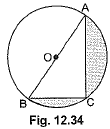Ans.
In ΔABC, ∠ACB = 90° (Angle in the semicircle)
∴ BC2 + AC2 = AB2
∴ BC2 = AB2 -AC2
= 169 - 144 = 25
∴ BC = 5 cm
Area of the shaded region = area of semicircle - area of right AABC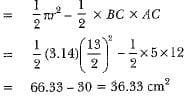Q.14. In Fig. 12.35, are shown two arcs PAQ and PBQ. Arc PAQ is a part of circle with centre 0 and radius OP while arc PBQ is a semi-circle drawn on PQ as diameter with centre M.
If OP = PQ = 10 cm show that area of shaded region is 25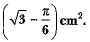[CBSE (Delhi) 2016]
Ans.
Since OP = PQ = QO
⇒ APOQ is an equilateral triangle
∴ ∠POQ = 60°
Area of segment PAQM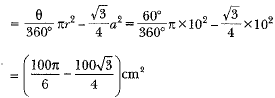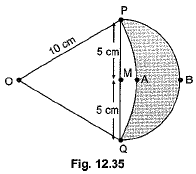Area of semicircle with M as centre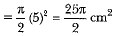Area of shaded region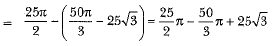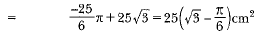Q.15. In figure, the boundary of shaded region consists of four semicircular arcs, two smallest being equal. If diameter of the largest is 14 cm and that of the smallest is 3.5 cm, calculate the area of the shaded region.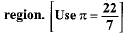[Foreign 2016]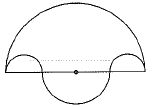Ans.
Given AD = 14 cm, AB = CD = 3.5 cm
∴ BC = 7 cm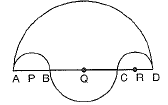Area of shaded region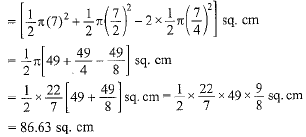Q.16. Find the area of the shaded region in Fig. 12.23, where a circular arc of radius 6 cm has been drawn with vertex 0 of an equilateral triangle ΔOAB of side 12 cm as centre.    [NCERT, CBSE (F) 2016 ]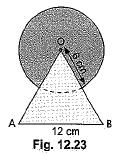Ans.
We have, radius of circular region = 6 cm and each side of ΔOAB = 12 cm.
∴ Area of the circular portion
= area of circle - area of the sector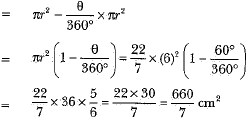Now, area of the equilateral triangle OAB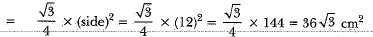∴ Area of shaded region = area of circular portion + area of equilateral triangle OAB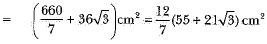Long Answer Type Questions

Q.1. A chord PQ of a circle of radius 10 cm subtends an angle of 60° at the centre of circle. Find the area of major and minor segments of the circle.    [Delhi 2017]
Ans.
Radius of the circle = 10 cm
Central angle subtended by chord AB = 60°
Area of minor sector OACB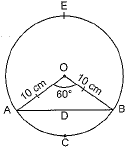Area of equilateral triangle OAB formed by radii and chord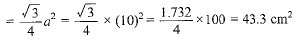∴ Area of minor segment ACBD
= Area of sector OACB - Area of triangle OAB
= (52.38 - 43.30) cm2 = 9.08 cm2
Area of circle = πr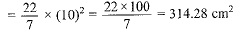∴ Area of major segment ADBE
= Area circle - Area of minor segment
= (314.28 - 9.08) cm2 = 305.20 cm2

Q.2. In the given figure, ΔABC is a right-angled triangle in which ∠A is 90°. Semicircles are drawn on AB, AC and BC as diameters. Find the area of the shaded region.     [Al 2017]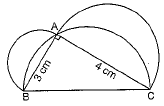Ans.
In right triangle ABC.
AB2 + AC2 = BC2
⇒ (3)2 + (4)2 = BC2 ⇒ 9 + 16 = BC2  ⇒ 25 = BC2
∴ BC = 5 cm
Now, Area of shaded region = Area of semicircle on side AB + area of semicircle on side AC - area of semicircle on side BC + area of ΔABC
Now, Area o f semicircle on side AB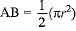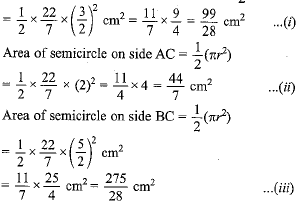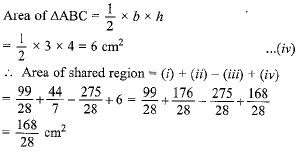Hence area of the shaded region = 6 cm2

Q.3. In Fig. 12.51, O is the centre of the circle with AC = 24 cm , AB = 7 cm and ∠BOD = 90°. Find the area of the shaded region.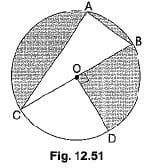[CBSE (AI) 2017]
Ans.
In right angle triangle ABC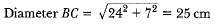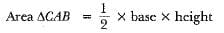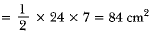Area of shaded region = area of semicircle - area of ΔCAB + area of quadrant BOD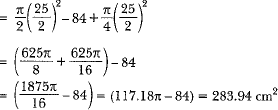Q.4. An elastic belt is placed around the rim of a pulley of radius 5 cm. (Fig. 12.46). From one point C on the belt, the elastic belt is pulled directly away from the centre O of the pulley until it is at P, 10 cm from the point O. Find the length o f the belt that is still in contact with the pulley. Also find the shaded area.  (Use π = 3.14 and √3 = 1.73)    [CBSE Delhi 2016]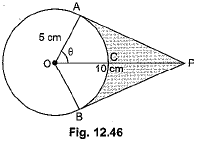Ans.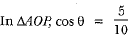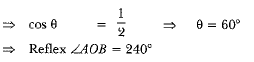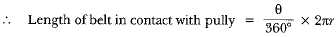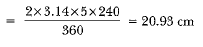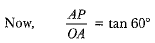PA = 5√S cm = BP (Tangents from an external point are equal)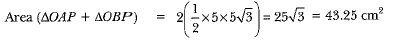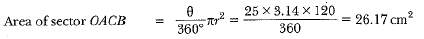Shaded area = 43 .25 - 26 .17 = 17.08 cm2

Q.5. In Fig. 12.47, a sector OAP of a circle with centre O, containing angle 0. AB is perpendicular to the radius OA and meets OP produced at B. Prove that the perimeter of shaded region is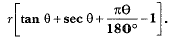[CBSE (AI) 2016]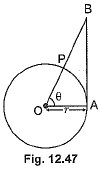Ans.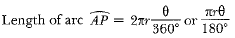In right ΔAOB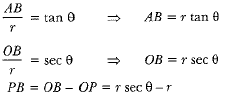Perimeter of shaded region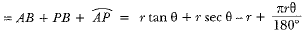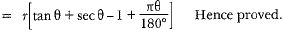Q.6. Find the area of the shaded region in Fig. 12.48, where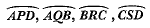are semicircles of diameter 14 cm, 3.5 cm, 7 cm and 3.5 cm respectively.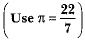[CBSE (E) 2016]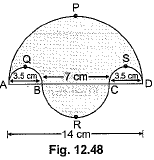Ans.
Area of shaded region =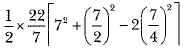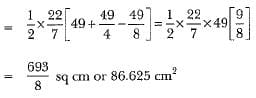Q.7.  In figure, ABCD is a trapezium with AB || DC, AB = 18 cm, DC = 32 cm and distance between AB and DC = 14 cm. If arcs of equal radii 7 cm with centres A, B, C and D have been drawn, then find the area of the shaded region.     [Foreign 2015]
Ans.
Area of shaded region = area of trapezium - (area of 4 sectors)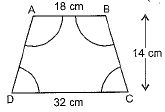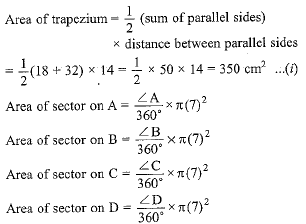Total area of all sector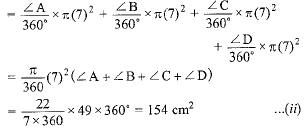[Sum of angles of a quadrilateral is 360°]
From (i) and (ii),
Area of shaded region = 350 - 154 = 196 cm2

Q.8. Find the area of the shaded region given in Fig.    [Delhi 2015]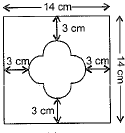Ans.
Side of the square = 14 cm
Area of the square = a2
= 142 - 196 cm2
Let radius of a semicircle=x
radius of two semicircles = 2x
side of inner square = diameter of semicircle = 2x
According to figure 2x + 2x = 8
4x = 8 ⇒ x = 2 cm
⇒ Side of inner square = 4 cm
Area of unshaded region = area of inner square + 4 (Area of a semicircle)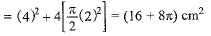∴ Area of shaded region = area of square - area of unshaded region
= (196-16-871) cm2 = (180-8π) cm2
= 180-8 x 3.14 = 180-25.12 = 154.88 cm2

The document Previous Year Questions: Areas Related to Circles - 2 Notes | Study Mathematics (Maths) Class 10 - Class 10 is a part of the Class 10 Course Mathematics (Maths) Class 10.
All you need of Class 10 at this link: Class 10

## Mathematics (Maths) Class 10

53 videos|369 docs|138 tests
 Use Code STAYHOME200 and get INR 200 additional OFF

## Mathematics (Maths) Class 10

53 videos|369 docs|138 tests

Track your progress, build streaks, highlight & save important lessons and more!

,

,

,

,

,

,

,

,

,

,

,

,

,

,

,

,

,

,

,

,

,

;